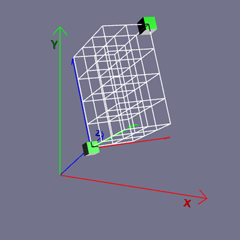# Position A Mesh

## Position

In order to fully understand all the ways to locate a mesh in the scene we need to know about frames of reference. When creating a world in a scene we are using a frame of reference in the World Space. In Babylon.js world space there are two horizontal axes, x and z, and a vertical y axis in a left handed system.

Each mesh in the scene also has its own Local Space. Meshes are created with their local space origin at the world space origin and with their local space x, y and z axes aligned with the x, y and z axes of the world space. Most, in not all, of the well known shapes have their local origin at the center of the mesh. The relative position of the local origin to the mesh shape depends on the data sets used in their construction.

Consider a mesh with its local origin in world space at (-1, 2, 1), that is mesh.position is at (-1, 2, 1); Babylon.js has a number of ways to reset the location a mesh. These are listed in the code below. The comments show the location of the local origin of the mesh in world space after each way has been applied.

The following are true for a mesh whether it has been rotated or not.

mesh.position = new Vector3(2, 3, 4);//(2, 3, 4)mesh.position.addInPlace(new Vector3(2, 3, 4)); //(-1 + 2, 2 + 3, 1 + 4) = (1, 5, 5)mesh.translate(new BABYLON.Vector3(2, 3, 4), 1, BABYLON.Space.WORLD); //(-1 + 2, 2 + 3, 1 + 4) = (1, 5, 5)
mesh.position.x = 2; //(2, 2, 1)mesh.position.y = 3; //(2, 3, 1)mesh.position.z = 4; //(2, 3, 4)
mesh.position.x += 2; //(-1 + 2, 2, 1) = (1, 2, 1)mesh.position.y += 3; //(1, 2 + 3, 1) = (1, 5, 1)mesh.position.z += 4; //(1, 5, 1 + 4) = (1, 5, 5)

Using the following methods the resulting position depends on the orientation of the mesh. It is not possible to give a resulting position without knowing the rotation of the mesh.

mesh.translate(new BABYLON.Vector3(2, 3, 4), 1, BABYLON.Space.LOCAL);mesh.setPositionWithLocalVector(new BABYLON.Vector3(2, 3, 4));mesh.locallyTranslate(new BABYLON.Vector3(2, 3. 4));

The image below shows how, using the last three methods above, how the orientation of the mesh affects its final position. The white grid lines lie in the current frame of reference, for the local space of the box mesh, which rotates with the mesh. The given vector (2, 3, 4) is applied in this frame of referenceNote: The vectors for position and setPositionWithLocalVector are position vectors. Those for translate, locallyTranslate and addInPlace are direction vectors.